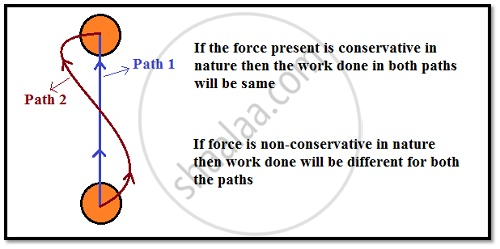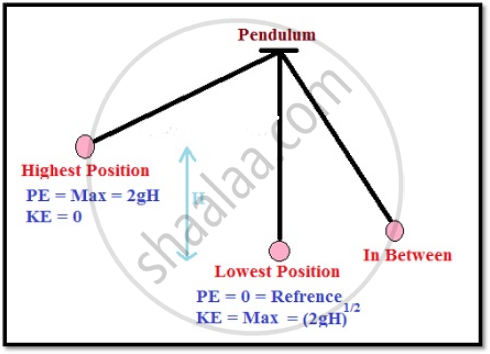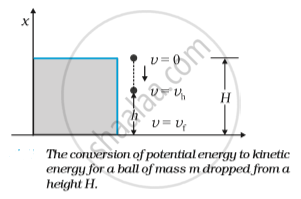Share

# The Conservation of Mechanical Energy

#### description

• Conservative Forces : Conservation of Mechanical Energy (Kinetic and Potential Energies)

#### notes

Conservative & Non-Conservative Forces

• Conservative forces are those for which work done depends only on initial and final points.
Example- Gravitational force, Electrostatic force.

• Non-Conservative forces are those where the work done or the kinetic energy did depend on other factors such as the velocity or the particular path taken by the object.
Example- Frictional force.

##The Conservation of Mechanical Energy

For conservative forces the sum of kinetic and potential energies of any object remains constant throughout the motion.
According to the quantum physics, mass and energy are not conserved separately but are conserved as a single entity called ‘mass-energy’.

• Mechanical Energy is the energy associated with the motion and position of an object.

• The quantity K +V(x), is called the total mechanical energy of the system.

• For a conservative force, ΔK = ΔW = F(x) Δx
Also, - V(x) = F(x) Δx

• This employs Δ(K+V) =0 for a conservative force.

• Individually the kinetic energy K and the potential energy V(x) may vary from point to point, but the sum is a constant.

Conservative Force:

• A force F(x) is conservative if it can be derived from a scalar quantity V(x) by the relation  : F(x) = - dv/dx

• The work done by the conservative force depends only on the end points.

• A third definition states that the work done by this force in a closed path is zero.

• The total mechanical energy of a system is conserved if the forces, doing work on it, are conservative.The above discussion can be made more concrete by considering the example of the gravitational force once again and that of the spring force in the next section. Fig. below depicts a ball of mass m being dropped from a cliff of height H.The total mechanical energies E0, Eh, and EH of the ball at the indicated heights zero (ground level), h and H, are
E_H = mgH
E_h = mgh + 1/2 mv_h^2
E_0 = (1/2) mv_f^2

The constant force is a special case of a spatially dependent force F(x). Hence, the mechanical energy is conserved. Thus
E_H = E_0
mgH = 1/2 mv_f^2
v_f= sqrt(2gH)
a result for a freely falling body.

Further,
E_H=E_h
which implies,
v_h^2 = 2g (H-h)
and is a familiar result from kinematics.

At the height H, the energy is purely potential. It is partially converted to kinetic at height h and is fully kinetic at ground level. This illustrates the conservation of mechanical energy.

S